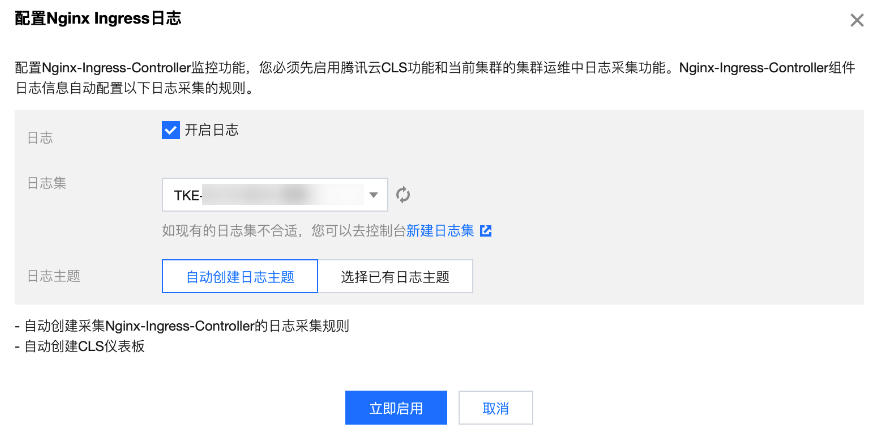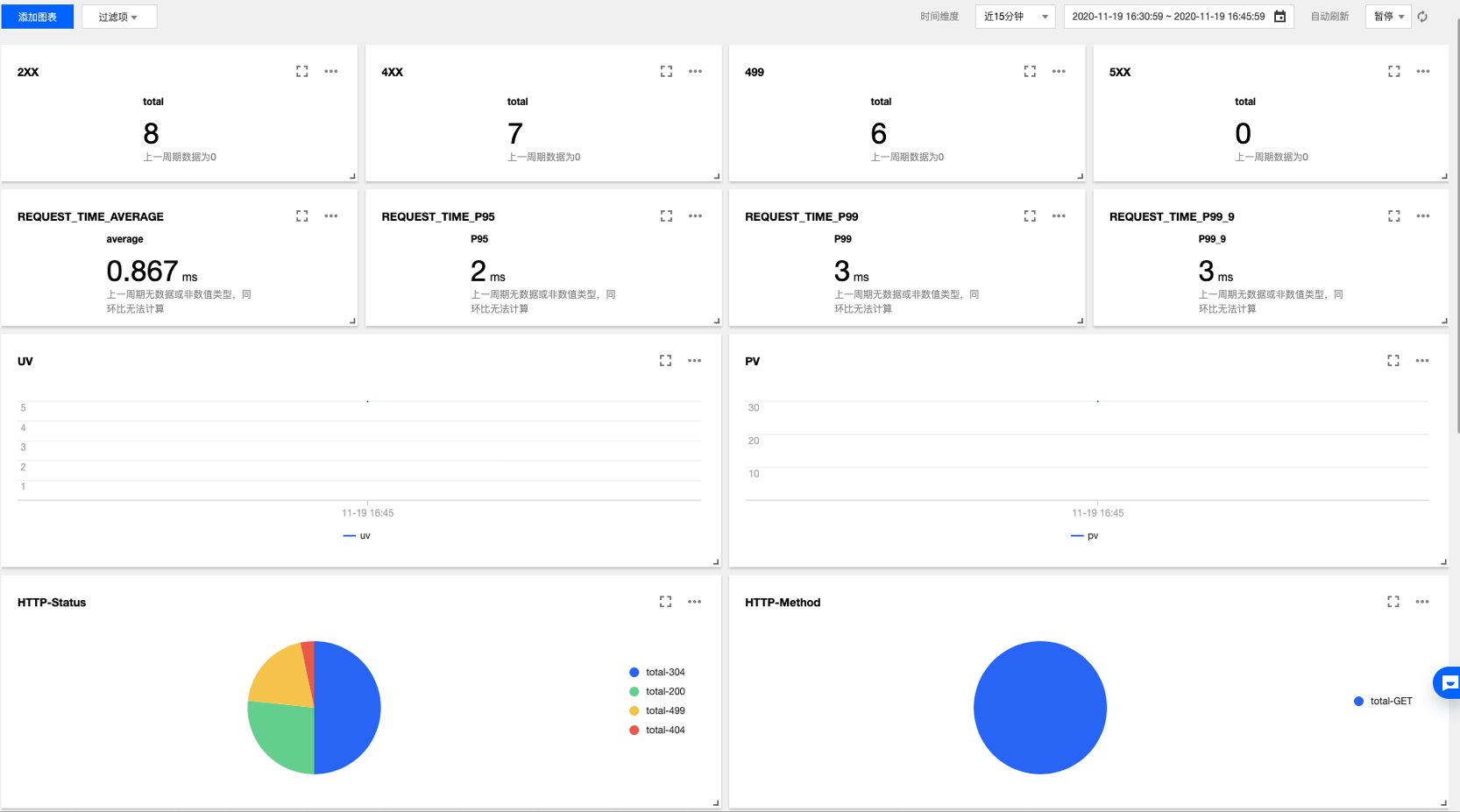## Nginx-ingress 日志基础

Nginx Controller 需要搜集以下日志并提供给用户：

• Nginx Controller 日志：重要。控制面日志，记录了 Nginx Controller 控制面的修改。主要用于控制面排障，例如用户错误配置 Ingress 模板导致同步未进行等。
• AccessLog 日志：重要。用户数据面日志，记录了用户的七层请求相关信息。主要用于提供给用户进行数据分析、审计、业务排障等。
• ErrorLog 日志：一般。Nginx 的内部错误日志。

## TKE Nginx-ingress 采集日志

### 采集日志步骤

1. 为目标集群 安装 Nginx-ingress 组件。
2. 在“组件管理”页面选择已安装的组件名称，进入组件详情页。
3. 日志监控页面中，选择日志配置右侧的重新设置。如下图所示：4. 在弹出的窗口中选择指定的日志集，如不制定将创建新的日志集。如下图所示：5. 单击立即启用即可完成日志采集配置。
注意：

日志服务具体计费规则和收费标准请参见 CLS 计费概述

### 采集日志指标

``````apiVersion: cls.cloud.tencent.com/v1
kind: LogConfig
name: nginx-ingress-test
resourceVersion: "7169042"
uid: 67c96f86-4160-****-****-f6faf8d544dc
spec:
clsDetail:
extractRule:
beginningRegex: (\S+)\s-\s(\S+)\s\[(\S+)\]\s(\S+)\s\"(\w+)\s(\S+)\s([^\"]+)\"\s(\S+)\s(\S+)\s\"([^"]*)\"\s\"([^"]*)\"\s(\S+)\s(\S+)\s\[([^\]]*)\]\s\[([^\]]*)\]\s\[([^\]]*)\]\s\[([^\]]*)\]\s\[([^\]]*)\]\s\[([^\]]*)\]\s(\S+)
keys:
- remote_user
- time_local
- timestamp
- method
- url
- version
- status
- body_bytes_sent
- http_referer
- http_user_agent
- request_length
- request_time
- proxy_upstream_name
- proxy_alternative_upstream_name
- upstream_response_length
- upstream_response_time
- upstream_status
- req_id
logRegex: (\S+)\s-\s(\S+)\s\[(\S+)\]\s(\S+)\s\"(\w+)\s(\S+)\s([^\"]+)\"\s(\S+)\s(\S+)\s\"([^"]*)\"\s\"([^"]*)\"\s(\S+)\s(\S+)\s\[([^\]]*)\]\s\[([^\]]*)\]\s\[([^\]]*)\]\s\[([^\]]*)\]\s\[([^\]]*)\]\s\[([^\]]*)\]\s(\S+)
logType: fullregex_log
inputDetail:
containerFile:
container: controller
filePattern: nginx_access.log
logPath: /var/log/nginx
namespace: default
kind: deployment
name: nginx-ingress-nginx-controller
type: container_file``````

### Nginx-ingress 日志仪表盘

TKE Nginx-ingress 开启日志采集功能将会自动为您创建一个标准的日志仪表盘，您也可以根据业务需要自行在 CLS 控制台配置图表。如下图所示：